Superluminal light attenuated by strong dispersion of complex refractive index
Ahmed Yonis Abdurahman, Nickolaevich Mal’nev Vadim, Mesfin Ali Belayneh†,Department of Physics, Addis Ababa University, P O Box 1176, Addis Ababa, Ethiopia

† Corresponding author. E-mail: belaynehmes@yahoo.com

Abstract
Abstract

The propagation of narrow packets of electromagnetic waves (EMWs) in frequency dispersive medium with the consideration of the complex refractive index is studied. It is shown that counting in the dispersion of the complex refractive index within the context of the conventional expression of the group velocity of narrow wave packets of EMWs propagating in a dispersive medium results in the appearance of additional constraints on the group velocity, which dictates that the physically acceptable group velocity can only be realized in the case of a negligible imaginary part of the group index. In this paper, the conditions that allow one to realize the physically acceptable group velocity are formulated and analyzed numerically for the relevant model of the refractive index of a system of two-level atoms in the optical frequency range. It is shown that in the frequency band where superluminal light propagation is expected, there is a strong dispersion of the refractive index that is accompanied with strong absorption, resulting in a strongly attenuated superluminal light.

1. Introduction

The studies of superluminal, backward, and slow narrow packets of light waves propagating in different media in the frequency range of strong dispersion of the refractive index have a long history. Currently, the question on the existence, physical meanings, and potential consequences of such waves has become a hot research topic and sometimes controversial. In this respect, there are two groups of researchers: those who support the existence of superluminal electromagnetic waves and those who reject such reports on grounds that it contradicts the special theory of relativity and consequently the so-called superluminal light has no physical meaning. In 1970 it was reported in Ref.  that Gaussian packets of electromagnetic waves can travel in dispersive media with group velocity exceeding the velocity of light in a vacuum with no restrictions whatsoever. Recently, the topic has been discussed theoretically in many papers again and even some have reported on the experimental realization of such waves in strongly dispersive media.

On the other hand, others have the view that the appearance of superluminal and negative group velocities of light cannot be attributed to a physical flow in the so-called superluminal region where there is strong dispersion of the refractive index that is accompanied by strong absorption. H. Tanka, et al. obtained negative group velocity as well as pulse velocity (group velocity) that can exceed the speed of light in a vacuum in Rb vapor, however they showed that at the frequency band where this velocity is attained the intensity of the transmitted pulse is reduced by strong dispersion and consequently concluded that the observed velocity is not the velocity of energy flow, i.e., group velocity. Similarly, it is reported that an electromagnetic wave (EMW) packet may travel at a superluminal velocity as well as negative group velocity, however the information carried by them is not superluminally transmitted and the information velocity carried by the wave front is still positive.

The interesting topic that needs further discussion concerns the superluminal light with group velocity Vg exceeding the speed of light in a vacuum. Such a value of Vg is commonly obtained with the help of the conventional formula that does not take into account the dispersion of the imaginary part of the refractive index. In this paper, we consider the evolutions of narrow packets of electromagnetic waves with the consideration of the dispersion of the complex refractive index n(ω). It is shown that this dispersion significantly distorts the shape of the Gaussian wave packet, which is demonstrated by introducing the imaginary part of the group index ng2 along with the conventional real group index ng1. The latter completely controls the group velocity with the help of the real part of n(ω) and its derivative with respect to the frequency ω. Our theoretical and numerical analyses show that the physically consistent group velocity Vg for a narrow packet of EMW can only be realized in the frequency bands where ng2ng1. By considering the relevant model of the complex refractive index of an assembly of two-level atoms, it is shown that in the frequency bands where the conventional formula of Vg gives the superluminal group velocity, the condition ng2ng1 is violated, confirming the fact that |Vg| > c has no physical meaning.

The rest of this paper is organized as follows. Section 2 is devoted to the study of the propagation of narrow packets of electromagnetic waves with the consideration of the dispersion of the imaginary part of the refractive index and the conditions on which the physically consistent group velocity can be realized are introduced. In Section 3, we consider the group indices and group velocity of light in an assembly of two-level atoms and determine the frequency bands where the physically acceptable group velocity is attained. The conclusions that are withdrawn from the results obtained in the paper are summarized in Section 4.

2. Evolution of narrow wave packets in dispersive medium

The profile of a Gaussian wave packet as a function of position x and time t propagating in a medium with dispersion can be obtained using the following equation: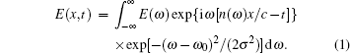Here, ω0 is the central frequency of the wave packet, E(ω) is its amplitude, σ is the width at frequency ω, and c is the speed of light in a vacuum. The refractive index of the medium n(ω) is a complex function of ω, given by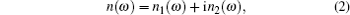where n1(ω) and n2(ω) are the real and imaginary parts of the refractive index, respectively.

For the realistic expressions of n(ω) and arbitrary σ, the integration in Eq. (1) is rather complex even in numerical calculation. However, it can be carried out analytically for narrow wave packets. For such cases, we may use the power series expansion of the complex refractive index (2) around ω0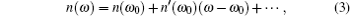where n′(ω0) is the first derivative of n(ω) evaluated at ω = ω0. For narrow wave packets the higher order terms in the expansion (3) can be ignored. In view of this, substitution of expansion (3) into Eq. (1) yields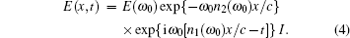Here, I denotes the following integral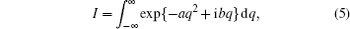where q = ωω0 and the parameters a and b are defined by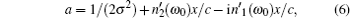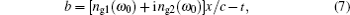with the introduction of the following notations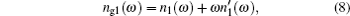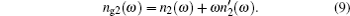The quantity ng1(ω) is known as the group index. Below, we introduce the imaginary part of the complex group index ng2(ω) along with the real one ng1(ω).

Equation (5) can be evaluated with the help of the Poisson integral. The result takes the following form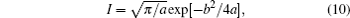provided that the real part of a is positive. It requires that the following condition must hold true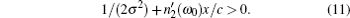Basically, equation (4) together with Eqs. (6)–(11) describes the evolution of the narrow wave packet propagating in the medium with a complex refractive index. To make the analysis of Eq. (4) more clear and simple, we consider a narrow wave packet traveling small distance x, when equation (6) reduces to a = 1/(2σ2). In view of this, equation (4) is simplified into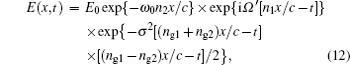where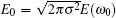and Ω′ = ω0 + σ2ng2x/c. The quantities n1, n2, ng1, and ng2 are to be evaluated at the central frequency ω0.

Let us compare Eq. (12) with the conventional result,[12,17] which follows from Eq. (12) by setting ng2 = 0 that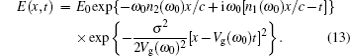Here, we introduce the group velocity Vg according to the well known relation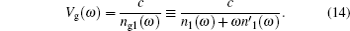Equation (13) shows that the narrow wave packet propagating in the dispersive medium with no consideration of the dispersion of the imaginary part of the refractive index can be presented as the envelope described by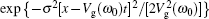of the plane wave exp{iω0[n1(ω0)x/ct]} moving with the group velocity Vg. The maximum of the envelope decreases with x, since n2(ω0) > 0 (the first exponent in Eq. (13)). This picture is simple and attractive.

However, the consideration of the dispersion of the imaginary part of the refractive index n2(ω) considerably changes this picture. Let us rewrite the last exponent in Eq. (12) in the form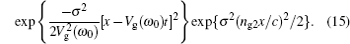In view of Eqs. (12), (13), and (15), we claim that it is possible to introduce Vg, defined by Eq. (14), having the physical meaning only in the frequency bands where Eq. (11) and the following inequalities hold true: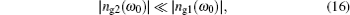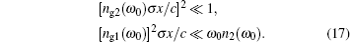Equation (17) provides a weak damping and can be satisfied for rather narrow wave packet σω0 and small traveling distance xc/[ω0n2(ω0)]. Inequality (16) is new and it imposes an important restriction on the conventional definition of Vg. The superluminal group velocity Vg > c is obtained from Eq. (14) in the frequency band where the following inequality holds true: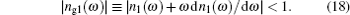In the next sections we focus on the consistency of Eqs. (16) and (18) for the relevant mathematical model of the refractive index of an assembly of two-level atoms.

3. Group index and group velocity of light in a system of two-level atoms

Let us consider a system consisting of weakly interacting two-level atoms with the bottom and upper levels represented by a and b, respectively. The refractive index of such weakly interacting two-level atoms is given by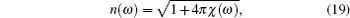where the susceptibility χ(ω) for a system in equilibrium is expressed as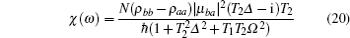with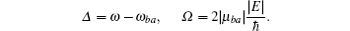Here, N is the density number of atoms, ωba > 0 is the transition frequency between the energy levels b and a, ρaa and ρbb are the diagonal components of the density matrix describing the difference in population between the levels a and b at thermal equilibrium, μba is the atom dipole matrix element, Δ is the detuning factor, T1 is the lifetime of the upper level, T2 is the characteristic time of dephasing dipole moment resulting in the transition line width 1/T2, Ω is the on-resonance Rabi frequency, and E is the amplitude of monochromatic electric field of the incident electromagnetic wave of frequency ω.

Below, we consider an equilibrium case when ρbb = 0 and ρaa = 1, that is, when the upper level is not populated. In the case of |χ| ≪ 1, which is consistent with the model, using Eqs. (19) and (20), we obtain the following expressions for the real and imaginary parts of the refractive index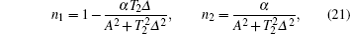where α = 2πN|μba|2T2/ħ and A2 = 1 + T1T2Ω2. Equation (21) is valid provided that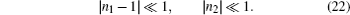For numerical analysis we evaluate α. Taking typical values, |μba| = 5.5 × 10−18 esu (for s → 3p transition of atomic sodium) and T2 = 32 ns, we obtain α = 6 × 10−15 N, where N is expressed in cm−3. It is clear that for N ≤ 1013 cm−3 and A ≥ 1, inequality (22) holds true.

The real and imaginary parts of the group index ng are obtained with the help of Eqs. (8), (9), and (21). The result becomes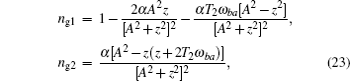where z = T2Δ. It is seen that far from the resonance frequency ω = ωba, where |z| ≫ 1, the approximation ng1 ∼ 1 and inequality |ng2| ≪ 1 can be satisfied by choosing the appropriate value of α, such that α ≪ 1. Therefore, inequality (16) for ng1 ∼ 1 and |ng2| ≪ ng1 holds true. At these frequencies the group velocity (14) has the physical meaning.

The frequencies in the vicinity of the resonance z = 0 are more interesting. To analyze the group indices in Eq. (23) at these frequencies, one has to take into account that T2ωba ≈ 107 (we set T2 ≈ 10−8 s and ωba ≈ 1015 rad/s). Keeping only the leading terms in Eq. (23), we obtain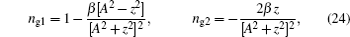where the parameter β = αT2ωba can be rather large though α is small.

Figures 13 show the curves of the real ng1 and imaginary ng2 parts of the group index versus z for β = 100 and different values of A, calculated using Eq. (24). Figure 1 shows the curve for A = 1. It corresponds to the case where the intensity of the incident wave |E|2 or the on-resonance Rabi frequency is small compared with the unit, i.e., T1T2Ω2 ≪ 1. The corresponding χ(ω) defined by Eq. (20) coincides with the first order susceptibility. In this case, the real part of the group index ng1 is of the known shape. For frequencies |z| ≫ 4, ng1ng2, consequently the corresponding group velocity Vg given by Eq. (14) has the physical meaning. However, for frequencies |z| ≤ 4, the situation completely changes. Here (with the exception of a narrow frequency band in the vicinity of z = 0) |ng2| > |ng1| so that the wave is strongly attenuated and inequality (16) is not valid. Therefore, the group velocity |Vg| > c has no physical meaning. However, in the frequency range where |ng1| < 1, equation (14) gives the group velocity corresponding to superluminal positive or negative light. In the vicinity of the exact resonance z = 0, ng2 ≈ 0 and inequality (16) holds true, giving Vg ≈ −c/100 that describes the negative slow light.

 Figure Option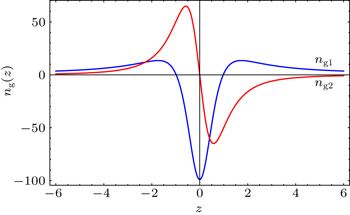Fig. 1. Curves of ng1 and ng2 versus z for β = 100 and A2 = 1.

Figure 2 shows the curves of ng1 and ng2 versus z for the same parameters as those in Fig. 1, but with A2 = 3, which corresponds to a rather intense optical field. In this case, the peaks of ng1 and ng2 decrease and become broad compared with Fig. 1. Again, one cannot ignore ng2 compared with ng1 for frequency |z| ≤ 6. In the vicinity of |z| ≈ 1.6, the conventional formula (14) gives superluminal group velocity. However, these velocities have to be discarded as they have no physical meanings since ng2 ∼ 10, near |z| = 1.6 resulting in a strongly attenuated wave. On the other hand, in the vicinity of the resonance z = 0, for frequency bands where |ng2| ≪ |ng1|, equation (16) is valid, giving Vg ≈ −c/30, corresponding to negative slow light.

 Figure Option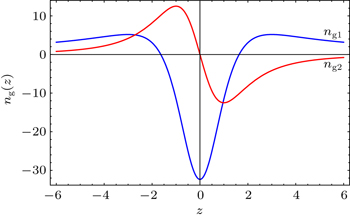Fig. 2. Curves of ng1 and ng2 versus z for β = 100 and A2 = 3.

Figure 3 shows the curves of ng1 and ng2 versus z for a much higher intensity of the incident optical wave with A2 = 5. One can observe that the peaks of the group indices become smaller and wider. This effect can be explained by the theory that the widening of the spectral line results in the presence of an intense radiation field. Moreover, the negative peak of ng2 becomes broader than those in Figs. 1 and 2. We can claim that the applied strong optical field of the incident wave broadens the frequency band where ng1 < ng2 and consequently the group velocity loses its physical meaning. For frequency far from the resonance, i.e., for z ≫ 6, the imaginary group index fast approaches to zero, being negative and equation (14) gives the physically admissible value of the group velocity |Vg| ≤ c.

 Figure Option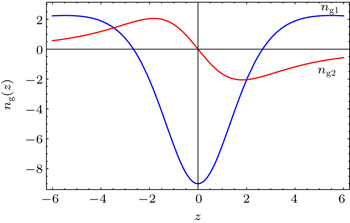Fig. 3. Curves of ng1 and ng2 versus z for β = 100 and A2 = 5.

It is necessary to note that again in the vicinity of the resonance z = 0, equation (14) results in a physically consistent group velocity corresponding to the slow negative light. The corresponding group index is obtained from the first expression of Eq. (24) and the group velocity is given by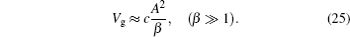This result is consistent with the curves of ng1 versus z depicted in Figs. 13.

4. Conclusions

The profile of a narrow wave packet propagating in a dispersive medium is analyzed with the help of the standard procedure but with the consideration of the dispersion of the imaginary part of the refractive index. In addition to the conventional group index ng1, it contains a new expression ng2, which corresponds to the imaginary part of the complex group index. This quantity significantly changes the profile of the wave packet compared with the conventional case, with ng2 = 0.

The main result of the paper is that the group velocity calculated using the conventional formula Vg = c/ng1 has the physically acceptable value only for frequency ω, where |ng2(ω)| ≪ |ng1(ω)|. We verify the validity of this inequality with the help of the refractive index of a typical two-level atom model at optical frequency for the equilibrium case and show that it is violated for the frequency range where the conventional formula of Vg gives superluminal group velocity |Vg| > c. That is, in the frequency domain where superluminal light is expected, the presence of strong absorption attenuates the wave and makes it impossible to realize the physical superluminal light.

On the other hand, in the vicinity of the resonant frequency where the imaginary part of the refractive index of an equilibrium system is large and results in strong absorption, the imaginary group index ng2 ≈ 0, which allows one to realize the physically consistent group velocity corresponding to the slow negative light with negative real group index |ng1| ≫ 1.

Reference
 1 Brillouin L1960Wave Propagation and Group VelocityNew YorkAcademic Press 2 Stenner M DGauthier D JNeifield M A 2003 Nature 425 695 3 Brunner NScarani VWegmuller MLegre MGisin N 2004 Phys. Rev. Lett. 93 203902 4 Garrett C G BMcCumber D E 1970 Phys. Rev. A 1 305 5 Boyd R WGauthier D J2002Progress in OpticsWolf EAmsterdamElsevier43497530 6 Milonni P W2005Fast Light, Slow Light, Left-Handed LightBristol and PhiladelphiaInstitute of Physics Publishing3567 7 Gauthier D JBoyd R W2007Photonics Spectra4182 8 Chu SWong S 1982 Phys. Rev. Lett. 48 738 9 Wang L JKuzmich ADogariu A 2000 Nature 406 277 10 Schweinsberg ALepeskin N NBigelow M SBoyd R WJarobo S 2006 Europhys. Lett. 73 218 11 Davidovich M V 2009 Physics-Uspekhi 52 415 12 Jackson J D1999Classical ElectrodynamicsNew YorkJohn Wiley & Sons, Inc.322339 13 Tasgm M E2012arXiv: 1204.5460v1 [physics.optics] 14 Tanka HNiurs HHayami KFurue SNakayama KKohmoto TKunitomo MFukuda Y 2003 Phys. Rev. A 68 053801 15 Kai CLong-An WYan-hua S 2004 Chin. Phys. Lett. 21 770 16 Ye DZheng GWang JWang ZQiao SHuangfu JRan L 2013 Sci. Rep. 3 1628 17 Boyd R W1992Nonlinear OpticsSan DiegoAcademic Press277292